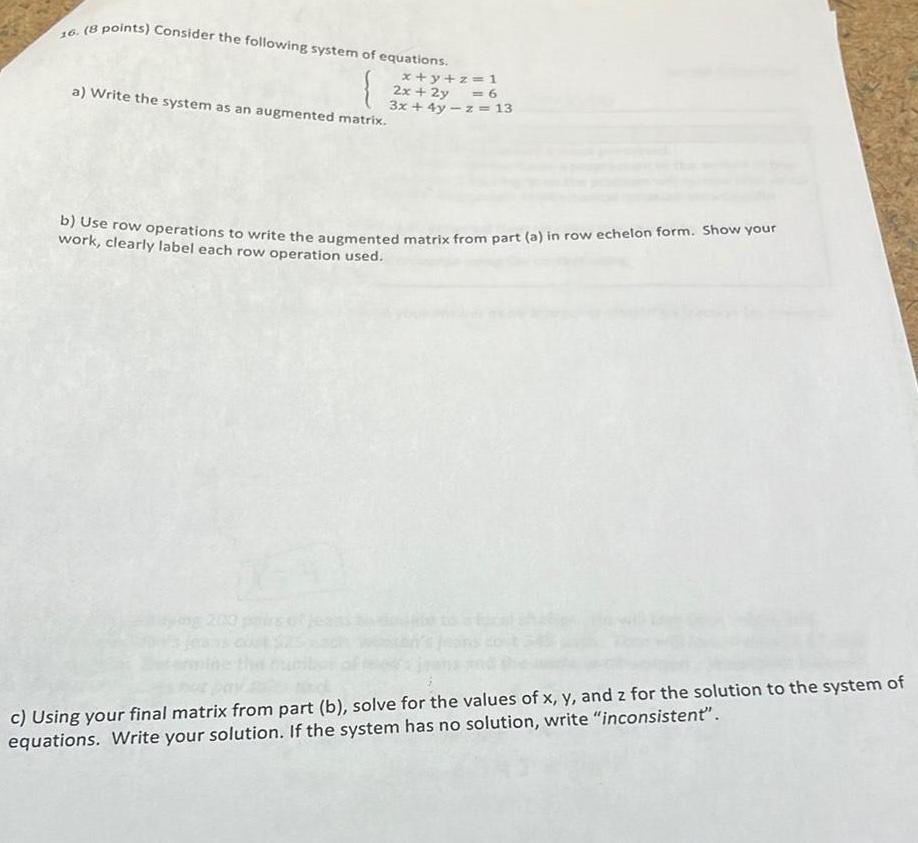Question:

# Consider the following system of equations. x+y+z=1 2x + 2y

Last updated: 8/14/2022Consider the following system of equations. x+y+z=1 2x + 2y = 6 3x + 4y -z = 13 a) Write the system as an augmented matrix. buse row operations to write the augmented matrix from part (a) in row echelon form. Show your work, clearly label each row operation used. c) Using your final matrix from part (b), solve for the values of x, y, and z for the solution to the system of equations. Write your solution. If the system has no solution, write "inconsistent'".